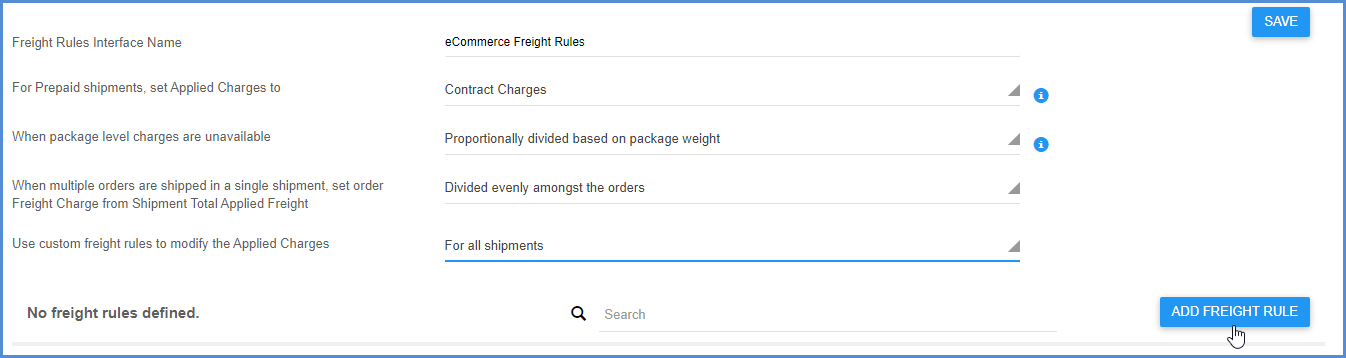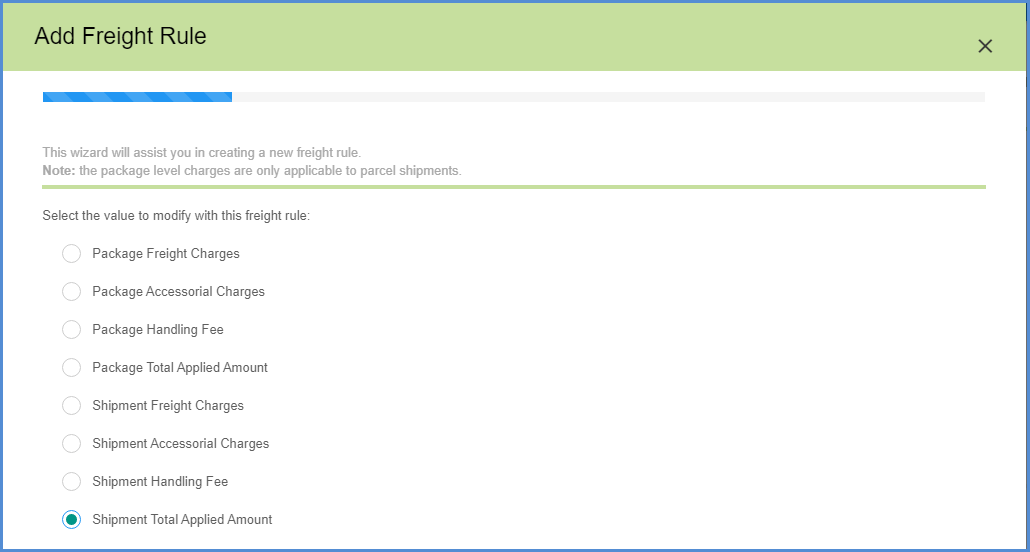As discussed in the Freight Rules Interfaces topic, when you select the option to “Use custom freight rules to modify the Applied Charges” for either prepaid or all shipments, you can use the freight rules wizard to create freight rules.### Value

The first step to defining a freight rule is to select the value that is going to be modified by the freight rule. This is the first screen of the freight rules wizard that appears after clicking the Add Freight Rule button.

Define the value to modify at the package or shipment level. You can select from Freight or Accessorial Charges, Total Applied Amount, or Handling Fee.### Type of Freight Rule

The next step is to define the type of rule, or the rule’s outcome on the value you selected above.###### Min/Max

This type of freight rule sets minimum an/or maximum boundaries to be applied to charges. Check the Minimum Amount and/or Maximum Amount check boxes to activate them. The Minimum Amount is the minimum charge allowed when this rule is applied. The Maximum Amount is the maximum amount that can be charged when this rule is applied.

• Value: Type a dollar amount into the field.
• Calculation: Select an existing calculation or create a new one by clicking New. The expanded calculation is displayed below the calculation field. For more information about creating a calculation, see Calculations.
###### Increase

This rule type adds a percentage, dollar amount, or calculated increase to be applied to the charges.

• Specific Amount: Type in the dollar amount by which to increase charges.
• Percentage: Type in a percentage by which to increase charges.
• Calculated Amount: Add a calculation that will define the amount of increase. Select an existing calculation or create a new one by selecting New. The expanded calculation is displayed below the calculation field.
###### Decrease

This rule type decreases charges by a percentage, dollar amount, or calculation. Click the radio button next to the option you want to use:

• Specific Amount: Type in the dollar amount by which to decrease charges.
• Percentage: Type in a percentage by which to decrease charges.
• Calculated Amount: Add a calculation that will define the amount of decrease. Select an existing calculation or create a new one by selecting New. The expanded calculation is displayed below the calculation field.
###### Fixed Amount

Set the value to a fixed amount per shipment, order in a shipment, or package; or, you can set the final value to a value from a StarShip shipment field.

###### Calculation

Set the value to the result of a user-defined calculation.

###### Do Not Write Back

Applied charges are not written back to the source interface.